# ISEE Lower Level Math : How to find the perimeter of a triangle

## Example Questions

1 2 3 4 6 Next →

### Example Question #1323 : Isee Lower Level (Grades 5 6) Mathematics Achievement

Find the perimeter of the following triangle: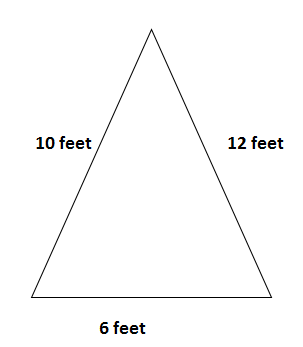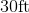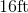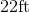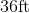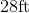Explanation:

To find the perimeter of a triangle, we will use the following formula: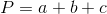where a, b, and c are the lengths of the sides of the triangle.

Now, given the trianglewe can see it has sides of length 10ft, 6ft, and 12ft.  Knowing this, we can substitute into the formula.  We get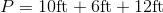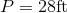### Example Question #371 : Plane Geometry

Find the length of one side of an equilateral triangle that has a perimeter of 27cm.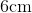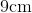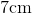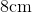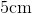Explanation:

The formula to find perimeter of an equilateral triangle is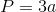where a is the length of one side.  We can multiply that by 3, because an equilateral triangle has 3 equal sides.  Now, to find the length of one side, we will solve for a.

So, we know the perimeter of the equilateral triangle is 27cm.  Knowing this, we can substitute.  We get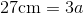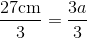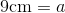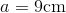Therefore, the length of one side of the equilateral triangle is 9cm.

### Example Question #1321 : Isee Lower Level (Grades 5 6) Mathematics Achievement

Use the following triangle to answer the question: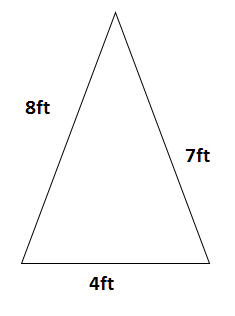Find the perimeter.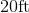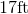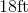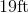Explanation:

To find the perimeter of a triangle, we will use the following formula:where a, b, and c are the lengths of the sides of the triangle.

Now, given the trianglewe can see that it has sides of length 8ft, 4ft, and 7ft.  So, we get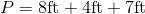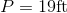### Example Question #373 : Plane Geometry

An equilateral triangle has a perimeter of 42 cm. What is the length of one of its sides?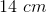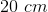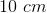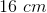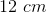Explanation:

By definition, an equilateral triangle is a triangle with three equal sides. That is, the length of each side of the triangle is going to be the same.

In this problem, we know that the perimeter (the sum of all the lengths) is 42 cm.

Side 1 + Side 2 + Side 3 = 42 cm.

Since the sides are equal, we can write the following equation.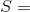one side of the triangle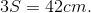To find the length of one side of the triangle, we would then divide 3 from both sides.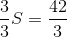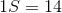Remember that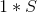is the same as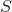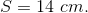1 2 3 4 6 Next →